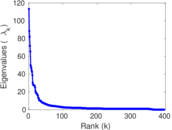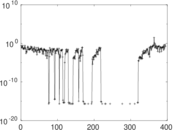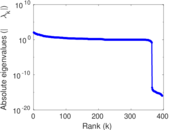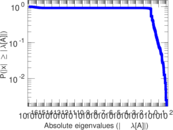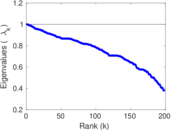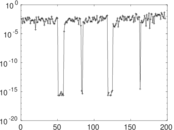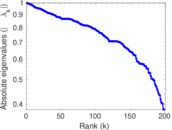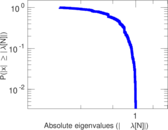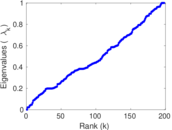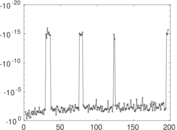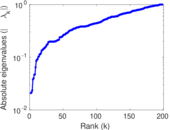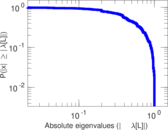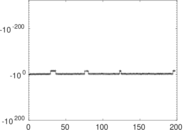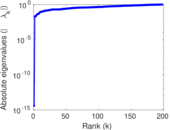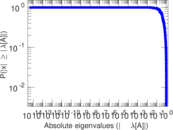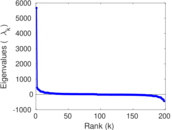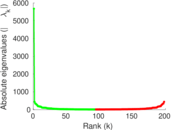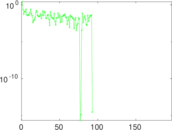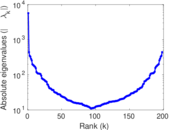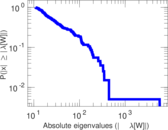# Wikibooks edits (bn)

This is the bipartite edit network of the Bangla Wikibooks. It contains users and pages from the Bangla Wikibooks, connected by edit events. Each edge represents an edit. The dataset includes the timestamp of each edit.

 Code `bbn` Internal name `edit-bnwikibooks` Name Wikibooks edits (bn) Data source http://dumps.wikimedia.org/ AvailabilityDataset is available for download Consistency checkDataset passed all tests Category Authorship network Dataset timestamp 2017-10-20 Node meaning User, article Edge meaning Edit Network formatBipartite, undirected Edge typeUnweighted, multiple edges Temporal dataEdges are annotated with timestamps

## Statistics

 Size n = 5,688 Left size n1 = 400 Right size n2 = 5,288 Volume m = 11,162 Unique edge count m̿ = 6,715 Wedge count s = 1,158,316 Claw count z = 216,001,006 Cross count x = 34,633,692,276 Square count q = 22,476 4-Tour count T4 = 4,828,502 Maximum degree dmax = 1,692 Maximum left degree d1max = 1,692 Maximum right degree d2max = 139 Average degree d = 3.924 75 Average left degree d1 = 27.905 0 Average right degree d2 = 2.110 82 Fill p = 0.003 174 64 Average edge multiplicity m̃ = 1.662 25 Size of LCC N = 5,328 Diameter δ = 12 50-Percentile effective diameter δ0.5 = 3.822 03 90-Percentile effective diameter δ0.9 = 5.849 36 Median distance δM = 4 Mean distance δm = 4.713 09 Gini coefficient G = 0.710 939 Balanced inequality ratio P = 0.214 657 Left balanced inequality ratio P1 = 0.096 577 7 Right balanced inequality ratio P2 = 0.317 416 Relative edge distribution entropy Her = 0.783 804 Power law exponent γ = 5.826 70 Tail power law exponent γt = 2.791 00 Tail power law exponent with p γ3 = 2.791 00 p-value p = 0.000 00 Left tail power law exponent with p γ3,1 = 1.771 00 Left p-value p1 = 0.003 000 00 Right tail power law exponent with p γ3,2 = 4.121 00 Right p-value p2 = 0.003 000 00 Degree assortativity ρ = −0.161 492 Degree assortativity p-value pρ = 1.807 01 × 10−40 Spectral norm α = 113.232 Algebraic connectivity a = 0.020 918 6 Spectral separation |λ1[A] / λ2[A]| = 1.280 50 Controllability C = 4,921 Relative controllability Cr = 0.871 128

## Plots

### Fruchterman–Reingold graph drawing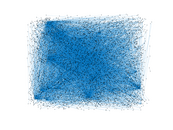### Degree distribution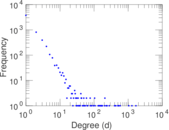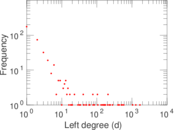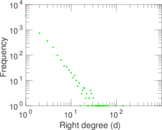### Cumulative degree distribution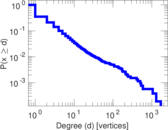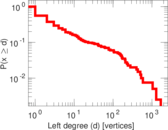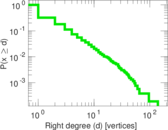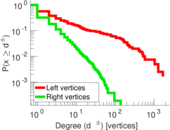### Lorenz curve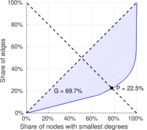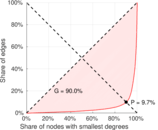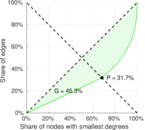### Spectral distribution of the adjacency matrix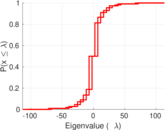### Spectral distribution of the normalized adjacency matrix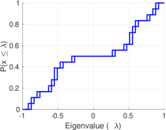### Spectral distribution of the Laplacian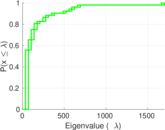### Spectral graph drawing based on the adjacency matrix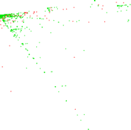### Spectral graph drawing based on the Laplacian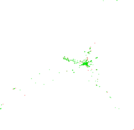### Spectral graph drawing based on the normalized adjacency matrix### Degree assortativity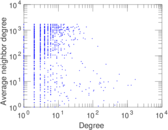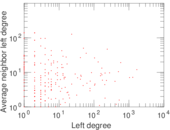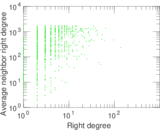### Zipf plot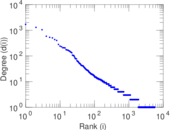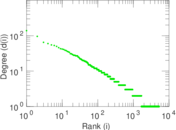### Hop distribution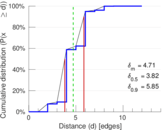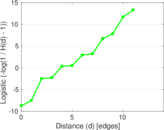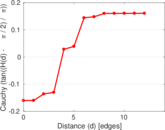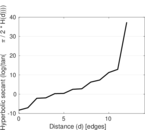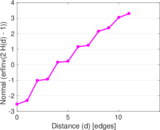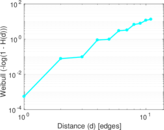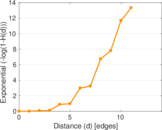### Double Laplacian graph drawing### Delaunay graph drawing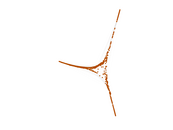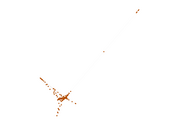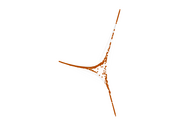### Edge weight/multiplicity distribution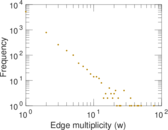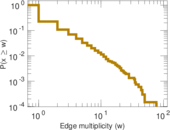### Temporal distribution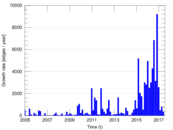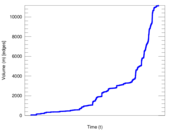### Temporal hop distribution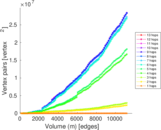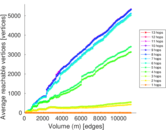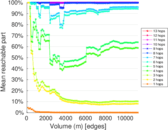### Diameter/density evolution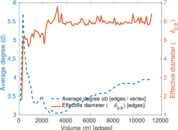### Matrix decompositions plots